Vibroengineering PROCEDIA

Published: 28 November 2019

# Measurement of damping properties of beeswax and cosmetic wax using Oberst beam method

Sachin Bhirodkar2
Venkatesham B3
1, 2, 3Department of Mechanical and Aerospace Engineering, Indian Institute of Technology, Hyderabad, Kandi, Telangana, 502285, India
Corresponding Author:
Venkatesham B
Views 369

#### Abstract

Damping treatment is a standard practice in many industries for controlling excessive vibration at resonance. Different damping materials are available for various engineering applications. Wax is one of them, and it’s mostly used in musical instruments. There are two types of wax are available namely beeswax (natural wax) and cosmetic wax. The aim of the present study is to measure damping properties of these two types of wax using Oberst Beam Method (OBM). The effects of damping material’s thickness and bare sample on damping loss factor are studied.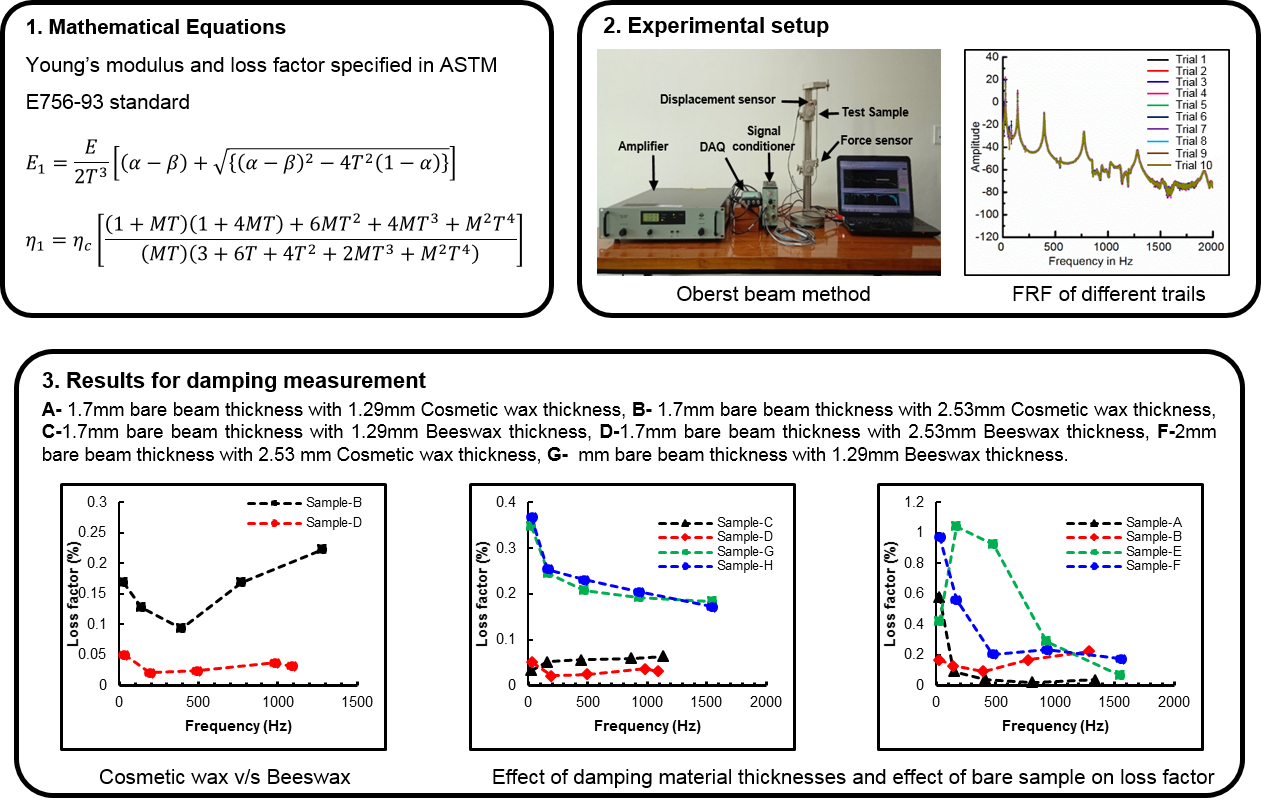#### Highlights

• Measured the damping properties of beeswax (natural wax) and cosmetic wax using Oberst Beam Method (OBM).
• In this study, two bare samples with eight composite beams (bare beam and damping material) are tested with a different thickness of the damping layer at room temperature.
• Study shows that damping properties of beeswax and cosmetic wax increase with increasing bare beam thickness and damping layer thickness.
• Cosmetic wax has a higher loss factor as compared to beeswax.

## 1. Introduction

Damping is a phenomenon in which mechanical energy is dissipated as heat in dynamical systems and is used for controlling excessive vibration at resonance. Reduction in the level of vibration amplitude depends on structure and damping material properties. Due to various applications of surface damping treatments in diverse engineering fields, a lot of research work is done on evaluation of damping properties using different techniques. Oberst Beam Method (OBM) is well-known and recommended by ASTM  and SAE standards for evaluation and measurement of material damping properties. Initial work on measurement and calculation of a damping loss factor of composite was done by Oberst and Frankenfeld in 1952 . Later in 1959, D. Ross et al., studied effect of damping on a plate flexural vibration by viscoelastic laminate . Using OBM, Jones  studied effect of temperature and frequency on the dynamic properties of damping material and showed that these parameters have a significant role in materials damping measurement.Wojtowicki et al.  proposed an alternative approach for measuring the damping properties of materials to avoid the experimental uncertainties and to improve the accuracy of the measurements. Instead of using conventional capacitive sensor, Jung et al.  used a Microflown velocity sensor for measuring loss factor, resonance factor and Young’s modulus of structural steel and polycarbonate. They compared measured results with standard OBM and analytically estimated results and proved that results from all the methods are in good agreement.

One of the naturally available damping materials is beeswax, which is produced by honeybees. They are obtained in two grades, one in yellow (natural) and the other is bleached beeswax (cosmetic wax). Both are used in different applications such as food, medical, skin care, musical instruments and cosmetic, etc. Most of research work was focused on characterization of structural properties of honeycomb panels inspired by a natural honeybee hive. However, authors have not come across literature available on damping properties of honeybee wax or cosmetic wax. Current research work is focused majorly on measurement of damping properties of beeswax and cosmetic wax. Also, studied the effect of damping material thickness on loss factor.

Damping properties can be measured either in frequency or time domain methods. In this study, a frequency-domain half bandwidth method is used to measure damping properties. Two bare samples with eight composite beams (bare beam and damping material) are tested with a different thickness of the damping layer at room temperature. Modal frequency of bare samples measured from OBM are corroborated with numerical and analytical results.

## 2. Theoretical review

This section briefly describes the ASTM standard method (resonant method) for calculating the loss factor, Young’s modulus of both bare and composite beam. These parameters are calculated based on measurement of Frequency Response Functions (FRF) of a cantilever beam.

### 2.1. Bare beam (Undamped)

Damping loss factor for a uniform beam of length $l$, thickens $H$ with density $\rho$ can be calculated by following expression, as specified in ASTM E756-93 standard :

1
$\eta =\frac{\mathrm{\Delta }{f}_{n}}{{f}_{n}},$

where, ${f}_{n}$ is resonance frequency of mode $n$ and $\mathrm{\Delta }{f}_{n}$ is half power bandwidth. Expression of Young’s modulus for bare beam as specified in ASTM E756-93 standard  is given by:

2
$E=\frac{12\rho {l}^{4}{f}_{n}^{2}}{{H}^{2}{C}_{n}^{2}},$

where, ${C}_{n}$ is coefficient for mode $n$ of the cantilever beam, $E$ is Young’s modulus of bare beam.

### 2.2. Beam with damping layer on a single side

In case of the composite beam (damping layer on a single side), expressions for Young’s modulus and loss factor specified in ASTM E756-93 standard are based on Ross-Kerwin-Ungar (RKU) equation :

3
${E}_{1}=\frac{E}{2{T}^{3}}\left[\left(\alpha -\beta \right)+\sqrt{\left\{{\left(\alpha -\beta \right)}^{2}-4{T}^{2}\left(1-\alpha \right)}\right\}\right],$
${\eta }_{1}={\eta }_{c}\left[\frac{\left(1+MT\right)\left(1+4MT\right)+6M{T}^{2}+4M{T}^{3}+{M}^{2}{T}^{4}}{\left(MT\right)\left(3+6T+4{T}^{2}+2M{T}^{3}+{M}^{2}{T}^{4}\right)}\right],$

where, ${E}_{1}$ is Young’s modulus of damping material (MPa), $M={E}_{1}/E$ is Young’s modulus ratio. $H$ is thickness of bare beam (m), ${H}_{1}$ is thickness of damping material (m), $T={H}_{1}/H$, thickness ratio. $\rho$ is density of bare beam (kg/m3), ${\rho }_{1}$ is density of damping material (kg/m3), $D={\rho }_{1}/\rho$ density ratio. $\alpha ={\left({f}_{c}/{f}_{n}\right)}^{2}\left(1+DT\right)$ and $\beta =4+6T+4{T}^{2}$. $\eta$ is loss factor of bare beam, ${\eta }_{1}$is loss factor of damping material, ${\eta }_{c}=\mathrm{\Delta }{f}_{c}/{f}_{c}$, loss factor of composite beam (bare beam and damping material), $\mathrm{\Delta }{f}_{c}$ is half power bandwidth for modes of composite beam (Hz), ${f}_{c}$ is resonance frequency for mode $n$ of composite beam (Hz), ${f}_{n}$ is resonance frequency for mode $n$ of bare beam (Hz). $l$ is length of bare beam (m), $I$ is moment of inertia of bare beam (m4), $A$ is area of bare beam (m2).

## 3. Measurement setup and procedure

### 3.1. Test samples details

In the current study, two bare samples of mild steel material with 7900 kg/m3 density with dimensions of 240 mm×20 mm×1.7 mm and 240 mm×20 mm×2 mm (length×width×thickness) are considered. Here, different thicknesses of bare samples are chosen to study effect of bare sample thickness on damping. Fig. 1 shows schematic of bare sample and with damping material as a layer. The root is a sample holding mechanism in Oberst beam method.

Fig. 1Schematic of test samples: a) bare sample, b) bare sample with damping layer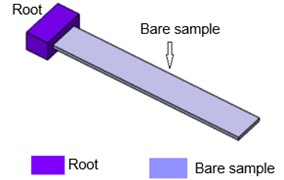a)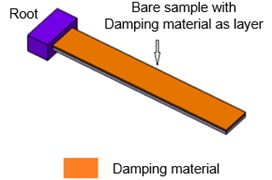b)

Natural wax is prepared by crushing honey bee hives (without honey) and then heated until it becomes a soft gel. It is applied to bare samples as a layer and curing is done at room temperature about 14 hours. For cosmetic wax (i.e. bleached wax which is available in the market), it is heated between 5to 10o C of above room temperature in wax heater and then applied to bare sample as a layer. The densities of these wax materials are calculated based on the measurement of weight by a volume ratio at room temperature and corresponding values are reported in Table 1. It also presents the details about test samples and damping materials. In present research, total eight test samples are studied (excluding bare samples) in damping test with a different combination of damping material (beeswax and cosmetic wax) and bare sample thickness. Details regarding these eight test sample’s notation are shown in Table 2.

Table 1Details of test bare samples and damping materials

 Parameters Details Parameters Details Boundary condition Clamped - Free Material tested Cosmetic wax Beam length (mm) 240 Beeswax Beam width (mm) 20 Density of bare beam 7900 kg/m3 Bare thickness (mm) 1.7 Cosmetic wax density 934 kg/m3 2 Beeswax density 873 kg/m3

Table 2Details of test samples notation in damping test

 Test sample Bare beam thickness ($H$) Damping material Damping material thickness (${H}_{1}$) A 1.7 mm Cosmetic Wax 1.29 mm B 1.7 mm Cosmetic Wax 2.53 mm C 1.7 mm Beeswax 1.29 mm D 1.7 mm Beeswax 2.53 mm E 2 mm Cosmetic Wax 1.29 mm F 2 mm Cosmetic Wax 2.53 mm G 2 mm Beeswax 1.29 mm H 2 mm Beeswax 2.53 mm

Table 3 shows parametric study to understand variation in damping property’s measurement with respect to damping materials, damping material thickness and bare sample thickness.

### 3.2. Oberst beam method (OBM)

Experimental test setup for Oberst beam is shown in Fig. 2(a). In this method, non-contact type magnetic force transducer (placed at a free end of the beam) and capacitive displacement transducer (placed near the clamped condition) are used. Input excitation to test sample is provided by force transducer, the response is measured by capacitive displacement transducer and is expressed in terms of Frequency Response Function (FRF). To avoid adverse effects of noncontact electromagnetic excitation, appropriate position of force and displacement transducer are obtained from different trial measurements . Necessary precautions are considered as per ASTM  standard during measurements. Here, a random signal is used as an input excitation and natural frequencies are measured based on the measured FRF by locating peaks. Damping properties are obtained using half-power method by locating the 3 dB reduction from peak amplitude of FRF.

Table 3Different parameters considered for the comparison study

 Case Comparisons Test samples considered Common parameters Damping material Damping material thickness Bare beam thickness I a Cosmetic wax v/s Beeswax A and C – 1.29 mm 1.7 mm b E and G – 2 mm c B and D – 2.53 mm 1.7 mm d F and H – 2 mm II a Cosmetic wax thickness A and B Cosmetic wax – 1.7 mm b E and F – 2 mm III a Natural wax thickness C and D Beeswax – 1.7 mm b G and H – 2 mm IV a Effect of bare beam thickness A and E Cosmetic wax 1.29 mm – b B and F 2.53 mm – c C and G Beeswax 1.29 mm – d D and H 2.53 mm –

Repeatability study of ten measurement trials has been conducted on 1.7 mm bare sample to verify consistency in ten measurements and corresponding results are shown in Fig. 2(b). It can be observed that there is good repeatability from all ten measurements.

Fig. 2a) Experimental setup for Oberst beam method (OBM), b) frequency response functions for different test trials measured using Oberst beam method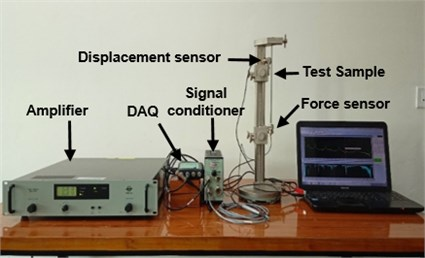a)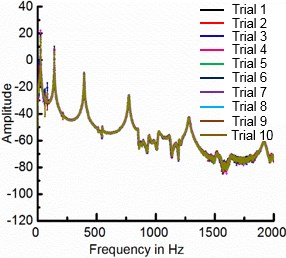b)

## 4. Results and discussion

### 4.1. Damping measurement of composite beam

Damping loss factor for composite beam (bare beam + damping material) of all eight samples (A to H) is measured using half-power bandwidth method. Cosmetic wax and beeswax composite loss factor are shown in Figs. 3(a) and (b), respectively. It can be observed that composite loss factor is increasing with respect to damping material thickness as well as bare beam thickness. This is due to more flexure in damping material. The deflection is more as damping layer is away from the neutral axis.

Fig. 3Composite loss factor with respect to frequency: a) Cosmetic wax, b) Beeswax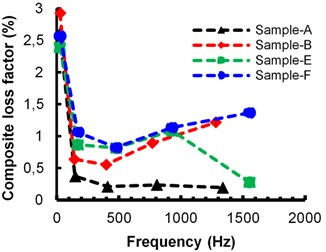a)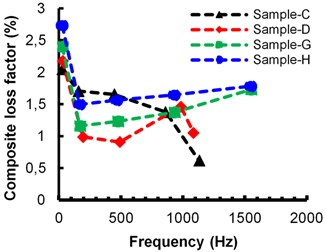b)

### 4.2. Cosmetic wax v/s Beeswax

To identify best damping material having higher loss factor out of cosmetic wax and beeswax, for bare beam thickness 1.7 mm samples B, D and for bare beam thickness of 2 mm samples F, H are considered for the study. These test samples consist of equivalent damping material thickness (2.53 mm) and same testing method is used. A comparison of measured cosmetic and beeswax properties are shown in Fig. 4. It is observed that cosmetic wax has higher loss factor compared to beeswax and is shown in Fig. 4(a) and (b).

Fig. 4Comparison of measured beeswax and cosmetic wax damping loss factor: a) 1.7 mm bare beam thickness, b) 2 mm bare beam thickness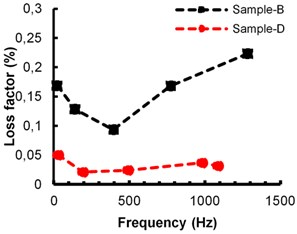a)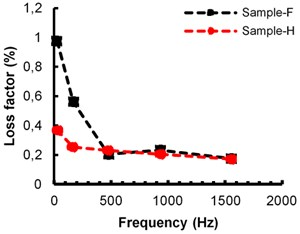b)

### 4.3. Effect of damping material thicknesses and effect of bare sample on loss factor

To understand the effect of damping material thickness on loss factor the following samples are considered. Cosmetic wax samples with 1.7 mm bare beam thickness of A (1.29 mm damping material), B (2.53 mm damping material) and 2 mm bare beam thickness of E (1.29 mm damping material), F (2.53 mm damping material). Beeswax samples with 1.7 mm bare beam thickness of C (1.29 mm damping material), D (2.53 mm damping material) and 2 mm bare beam thickness of G (1.29 mm damping material), H (2.53 mm damping material). These sample result plots are shown in Fig. 5. Based on this study, it is observed that loss factor of damping material increases with increasing thickness of cosmetic wax and beeswax as shown in Fig. 5(a) and (b), respectively.

Beeswax samples of D (1.7 mm bare beam) and H (2 mm bare beam) and Cosmetic wax samples of B (1.7 mm bare beam), F (2 mm bare beam) are considered to understand the effect of bare sample thickness on material loss factor and corresponding results are shown in Fig. 5(a, b). For both the damping materials, damping thickness of 2.53 mm is considered. It can be observed from Fig. 5 that if thickness of bare sample increases then loss factor of damping material also increases without changing damping material thickness. The flexure deflection of damping layer increases as outer layer distance also increases from neutral axis for thicker bare beams.

Fig. 5Effect on loss factor due to thickness of damping material: a) cosmetic wax, b) beeswax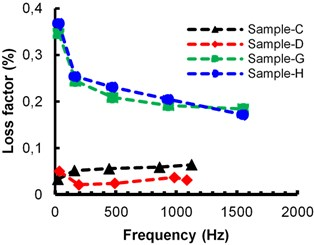a)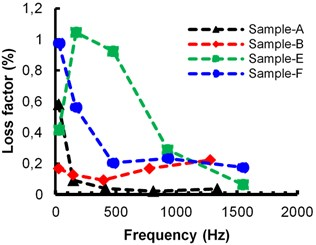b)

## 5. Conclusions

In the present study, measurement of damping properties of beeswax (natural wax) and cosmetic wax has been discussed. A repeatability study was done for measuring natural frequencies, and loss factor of the bare beam. An extensive parametric study was conducted. The present study shows that damping properties of beeswax (natural wax) and cosmetic wax increase with increasing bare beam thickness and damping layer thickness. Additionally, out of beeswax and cosmetic wax, cosmetic wax has a higher loss factor as compared to beeswax.